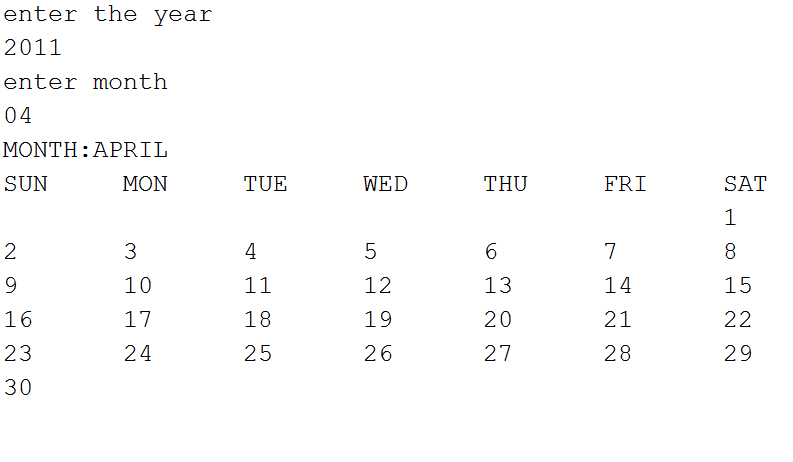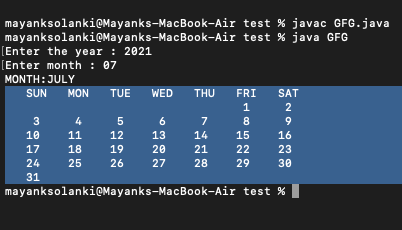Related Articles

# Java Program to Generate Calendar of Any Year Without calendar.get() Function

• Difficulty Level : Expert
• Last Updated : 19 Aug, 2021

Java program for generating the calendar of any desired year and month let us first go through an illustration before landing upon logic and procedural part.

Illustration:

Say the user wants to get the calendar of April 2011. Then, he is required to enter the year along with the month as integers and the output would return the desired month’s calendar for the respective year in a proper format.Procedure:

Step 1: Take the year and the month as integer inputs from the user

Step 2: Create 2 arrays as follows, one for storing days and the other for storing the months, as per proper order.

```String day[]   = { "SUN","MON","TUE","WED","THU","FRI","SAT" } ;
String month[] = { "JANUARY","FEBRUARY","MARCH","APRIL","MAY","JUNE","JULY","AUGUST","SEPTEMBER","OCTOBER","NOVEMBER","DECEMBER" } ; ```

Step 3: Initialize a counter variable and three variables, each for the day, month, and year as 1, and a separate array for storing the different combinations of days on which months can be found. Eg. 31,30,29

`int ar[] = { 31,29,31,30,31,30,31,31,30,31,30,31 } ;`

Step 4: Check the leap year condition and re-initialize values for the above array.

```if(y%4==0&&y%100!=0||y%100==0)
ar=29;                       // if the year is a leap year then store 29 for the month of february
else
ar=28;                       // else 28 ```

Step 5: Increment year count as month count reaches 12 and increment month count as day count reaches a value greater than that present in the array for the respective index

Step 6: Print the result.

Implementation:

Example

## Java

 `// Java Program to Generate Desired Calendar``// Without calendar.get() function or``// Inputting the Year and the Month`` ` `// Importing required classes``import` `java.io.*;``import` `java.util.Scanner;`` ` `// Main class``public` `class` `GFG {`` ` `    ``// Main driver method``    ``public` `static` `void` `main(String a[])``    ``{`` ` `        ``// Reading input by creating object of Scanner class``        ``Scanner sc = ``new` `Scanner(System.in);`` ` `        ``// Display message only``        ``System.out.print(``"Enter the year : "``);`` ` `        ``// Reading integer input value``        ``int` `yy = sc.nextInt();`` ` `        ``// Display message only``        ``System.out.print(``"Enter month : "``);`` ` `        ``// Reading integer input value``        ``int` `mm = sc.nextInt();`` ` `        ``int` `d = ``1``;``        ``int` `m = ``1``;``        ``int` `y = ``1``;``        ``int` `dy = ``1``;`` ` `        ``// Storing data and months as input``        ``String day[] = { ``"SUN"``, ``"MON"``, ``"TUE"``, ``"WED"``,``                         ``"THU"``, ``"FRI"``, ``"SAT"` `};``        ``String month[]``            ``= { ``"JANUARY"``, ``"FEBRUARY"``, ``"MARCH"``,``                ``"APRIL"``,   ``"MAY"``,      ``"JUNE"``,``                ``"JULY"``,    ``"AUGUST"``,   ``"SEPTEMBER"``,``                ``"OCTOBER"``, ``"NOVEMBER"``, ``"DECEMBER"` `};`` ` `        ``// Custom array as input``        ``int` `ar[] = { ``31``, ``29``, ``31``, ``30``, ``31``, ``30``,``                     ``31``, ``31``, ``30``, ``31``, ``30``, ``31` `};`` ` `        ``// Till condition holds true``        ``while` `(``true``) {`` ` `            ``if` `(d == ``1` `&& m == mm && y == yy) {``                ``break``;``            ``}`` ` `            ``if` `(y % ``4` `== ``0` `&& y % ``100` `!= ``0``                ``|| y % ``100` `== ``0``) {``                ``ar[``1``] = ``29``;``            ``}`` ` `            ``else` `{``                ``ar[``1``] = ``28``;``            ``}``            ``dy++;``            ``d++;`` ` `            ``if` `(d > ar[m - ``1``]) {``                ``m++;``                ``d = ``1``;``            ``}`` ` `            ``if` `(m > ``12``) {``                ``m = ``1``;``                ``y++;``            ``}`` ` `            ``if` `(dy == ``7``) {``                ``dy = ``0``;``            ``}``        ``}`` ` `        ``int` `c = dy;`` ` `        ``if` `(y % ``4` `== ``0` `&& y % ``100` `!= ``0` `|| y % ``400` `== ``0``) {``            ``ar[``1``] = ``29``;``        ``}``        ``else` `{``            ``ar[``1``] = ``28``;``        ``}`` ` `        ``// Print the desired month of input year``        ``System.out.println(``"MONTH:"` `+ month[mm - ``1``]);`` ` `        ``for` `(``int` `k = ``0``; k < ``7``; k++) {``            ``System.out.print(``"   "` `+ day[k]);``        ``}`` ` `        ``System.out.println();`` ` `        ``for` `(``int` `j = ``1``; j <= (ar[mm - ``1``] + dy); j++) {``            ``if` `(j > ``6``) {``                ``dy = dy % ``6``;``            ``}``        ``}`` ` `        ``int` `spaces = dy;``        ``if` `(spaces < ``0``)``            ``spaces = ``6``;`` ` `        ``// Printing the calendar``        ``for` `(``int` `i = ``0``; i < spaces; i++)``            ``System.out.print(``"      "``);``        ``for` `(``int` `i = ``1``; i <= ar[mm - ``1``]; i++) {``            ``System.out.printf(``" %4d "``, i);`` ` `            ``if` `(((i + spaces) % ``7` `== ``0``)``                ``|| (i == ar[mm - ``1``]))``                ``System.out.println();``        ``}``    ``}``}`

Output:Attention reader! Don’t stop learning now. Get hold of all the important Java Foundation and Collections concepts with the Fundamentals of Java and Java Collections Course at a student-friendly price and become industry ready. To complete your preparation from learning a language to DS Algo and many more,  please refer Complete Interview Preparation Course.

My Personal Notes arrow_drop_up